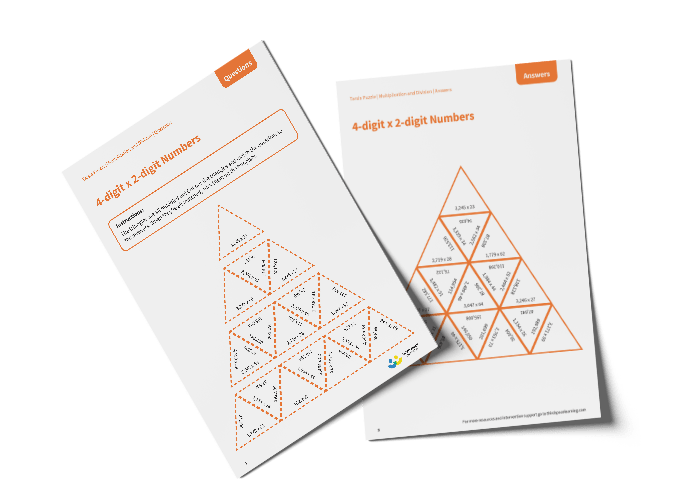# Tarsia Puzzle 4-digit Multiplied by 2-digit Numbers (Year 6)

The triangles in the tarsia puzzle have all been mixed up. In this 4-digit multiplied by 2-digit numbers tarsia puzzle, pupils will need to match the multiplication question to the correct answer to create one large triangle.

This Year 6, 4-digit multiplied by 2-digit numbers tarsia puzzle worksheet contains:

• One question sheet
• One template sheet

Year 6

Multiplication